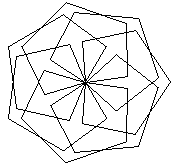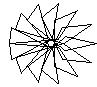#Thomas S. Ferguson

Thomas S. Ferguson is a Professor in the Department of Mathematics and the Department of Statistics at the University of California at Los Angeles.

UCLA Department of Mathematics
520 Portola Plaza, Suite 6363
Los Angeles, CA 90095-1555
e-mail: tom@math.ucla.edu

##Some recent papers:

• "Testing equality of players in a round-robin tournament", with F. Thomas Bruss, The Mathematical Scientist, Vol. 43 No. 2, Dec. (2018), 125-136. (pdf version.)
• "House-Hunting Without Second Moments", with Michael J. Klass, Sequential Analysis, 29, (2010), 236-244. (pdf version 148K.)
• "The Sum-the-Odds Theorem with Application to a Stopping Game of Sakaguchi", Mathematica Applicanda 44(1), (2016), 45-61. (pdf version 176K.)
• "The Endgame in Poker", with Chris Ferguson, in Optimal Play - Mathematical Studies of Games and Gambling, Stewart Ethier and William R. Eadington, eds. (2007) 79-106. Institute for the Study of Gambling and Commercial Gaming. (pdf version 236K.)
• "U(0,1) Two-Person Poker Models", with Chris Ferguson and Céphas Gawargy, Game Theory and Applications, 12 (2007), 17-37, Nova Sci. Publ., N.Y. (pdf version 132K.)
• "On the Borel and von Neumann Poker Models", with Chris Ferguson, Game Theory and Applications, 9 (2003), 17-32, Nova Sci. Publ., N.Y. (pdf version 152K.)
• "An Optimal Strategy for Sequential Classification on Partially Ordered Sets", with Curtis Tatsuoka, Statistics Probability and Letters, 68, (2004) 161-168. (pdf version 124K.)
• "Inverting Dirichlet Tessellations", with Rick Schoenberg and Cheng Li, The Computer Journal, 46 (2003), 76-83. (pdf version 400K.)
• "Notes on a Stochastic Game with Information Structure", with Lloyd Shapley and Robert Weber, International J. Game Theory, 31 (2003), 223-228. (pdf version 204K.)
• "Toetjes na", with Christian Genest, Mathematical Statistics and Applications: Festschrift for Constance van Eeden, Marc Moore, Sorana Froda and Christian Léger, Editors, IMS Lecture Notes/Monograph Series, 42 (2003), 169-184. (Abstract, pdf version, 175K)
• "Selection by Committee", in Advances in Dynamic Games, A.S. Nowak and K. Szajowski eds., (2004) 183-188, Birkhäuser. By mistake, the published version is an earlier version without proofs. The correct version is here. (pdf version, 140K).
• "Sequential Classification on Partially Ordered Sets", with Curtis Tatsuoka, J. R. Statist. Soc. B, 65, (2003)143-157. (Abstract, pdf version 184K).
• "On a Rao-Shanbhag Characterization of the Exponential/Geometric Distribution", Sankhya Series A, 64, (2002) 246-255. (Abstract, pdf version 100K).
• "High Risk and Competitive Investment Models", with F. Thomas Bruss, Ann. Applied Prob., 12 (2002), 1202-1226. (Abstract, pdf version 220K).
• "Another Form of Matrix Nim", Electronic J. of Combinatorics 8 (No. 2) (2001), #R9. (Abstract, pdf version 88K).
• "Kendall's tau for Serial Dependence", with Christian Genest and Marc Hallin, Canadian J. Statist., 28 (2000) 587-604. (Abstract, pdf version 200K).
• Some less recent papers.
• Unpublished papers.

##Problems

1. The joint distribution of two Bernoulli random variables, X and Y, is determined by the 2 by 2 matrix of probabilities, p(i,j) = P(X=i,Y=j) for i=0,1 and j=0,1. Suppose the (prior) distribution of (p(0,0),p(0,1),p(1,0),p(1,1)) is uniform on the set {p(0,0)>0, p(0,1)>0, p(1,0)>0, p(1,1)>0, p(0,0)+p(0,1)+p(1,0)+p(1,1)=1}. (This is the Dirichlet distribution with parameters 1,1,1,1.) Find the conditional distribution of the p(i,j) given that X and Y are independent (i.e. given P(X=i,Y=j) = P(X=i)P(Y=j)). Need a hint?

2. A fair coin is tossed until it comes up heads and the number, X, of tails before the first heads appears is recorded. Then two slips of paper are taken and the number 3 to the power X is written on one of the slips and 3 to the power X+1 is written on the other. One of these two slips is chosen at random (probability 1/2 each) and given to you. It will have one of the numbers 1, 3 ,9, 27, 81, etc. written on it. After observing this number, call it z, you are given the option of exchanging your slip for the other, unseen slip. Then you are paid in dollars the number on the slip you possess. For which values of z would you make the exchange? For which values of z would you make the exchange if you had to pay a 10% commission to the broker who effects the exchange? Need a hint?

3. Kriegspiel is the game of chess in which the players are not allowed to see the moves of the opponent. Instead, there is a "referee" who keeps track of the each move on his own private board and tells the players if an attempted move is legal. If an attempted move is legal, the player must play it; otherwise, the player may try again. In addition, the referee announces all captures and whether the captured object is a piece or a pawn. In the RAND rules, the referee also announces checks, and possible captures by a pawn.

The question is: Can a blind person, hearing only the announcements of the referee, deduce a mate for one of the players? Need a hint?

4. Two positive integers are chosen. The sum is revealed to logician A, and the sum of the squares is revealed to logician B. Both A and B are given this information and the information contained in this sentence. The conversation between A and B goes as follows: B starts.

B: "I can't tell what the two numbers are."
A: "I can't tell what the two numbers are."
B: "I can't tell what the two numbers are."
A: "I can't tell what the two numbers are."
B: "I can't tell what the two numbers are."
A: "I can't tell what the two numbers are."
B: "Now I can tell what the two numbers are."

What are the two numbers? Need a hint?

5. The outcome of a certain experiment results in three (possibly dependent) random variables, X, Y, Z, each taking values either zero or one. (So there are 8 possible outcomes to the experiment.) Is it possible to set up such an experiment so that

P(X = Y) + P(X = Z) + P(Y = Z) < 1 ?

Need a hint?